Example Questions

Example Question #1 : Applying Percents To Word Problems

Portia purchased a laptop for $480, but after checking the merchant's website realized that she had been overcharged by 20%. By how much, in dollars, was she overcharged? Possible Answers:$96

$48$24

$80 Correct answer:$80

Explanation:

In any percent problem, it is crucial that you know exactly which value the percent is to be taken of. Here Portia is overcharged by 20%, meaning that she paid 20% more than the item should have cost. That means that the amount she paid, $480, equals 20% more than she should have paid: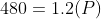, where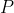is the price she should have paid. To solve for, divide both sides by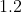: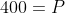This means that she should have paid$400 but instead paid $480, so she overpaid by$80.

Note that a common mistake on percent problems is taking the percent of the wrong value: here you might be tempted to simply take 20% of 480, for example. The SAT test makers know that, and will often set up problems so that you're given the result of the percent change (here the result is Portia paying $480) and need to work your way back to the start value as you did here. Always pause to ensure that you know which value (or variable) gets multiplied by the percent change. Example Question #2 : Applying Percents To Word Problems Over the course of a single week, the price of a company’s stock rose by 10%. If the following week the company’s stock rose by 10% of the new price, by what percent did the stock increase over the two week period? Possible Answers: 120% 19% 21% 20% Correct answer: 21% Explanation: This problem rewards students who do the work rather than just looking at a problem and going with what “looks right.” Whenever you see a problem that asks you to find the percent difference between two unknowns, you can probably pick your own numbers to make the problem easier since the problem is asking for a relationship rather than a value. In this case, since you are dealing with percents, it is a good idea to start with the number 100. If you increase 100 by 10%, you will get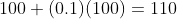after the first increase. During the second week, you are told, the price of the stock increases by 10% of the new value. Be careful here to take the percent of the correct value, 110, rather than the original value. If you increase 110 by 10%, you get: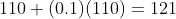. To find the percent increase from there, you find the difference and then divide by the original before multiplying by 100: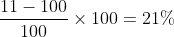Example Question #3 : Applying Percents To Word Problems A store buys a shirt for$60, then marks it up by 50%, then offers customers a 20% discount off the marked up price. What price do consumers pay?

$78$72

$90$108

$72 Explanation: Remember that when you are dealing with taking the percent of a number and then taking the percent of the result, that you must be careful to take the percent of the correct number. In this case, that means increase$60 by 50% and then taking 20% off that new number. What you cannot do is either take off 20% of 60 or just increase 60 by 30% rather than doing each step in order. Doing either will lead to a trap that the Testmaker has left for you.

The first step you’re given is to increase $60 by 50%. This is the same as taking 150% of$60, or as multiplying it by 1.5. If you do this you get;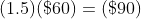From there, you now need to find what 20% off 90 is in order to get your solution. You can do this by first finding 10% of 90 by moving the decimal point one place to the left to get 9 and then doubling it to get 18.

Example Question #7 : Applying Percents To Word Problems

After the first week of training for an upcoming race, Dinesh begins the second week of his training program by increasing his weekly running mileage by 50%. After three weeks, he catches a cold and has to reduce his current weekly mileage by 40% for the final week of training. By what percent did Dinesh's weekly mileage change from the first week to the final week?

10% decrease

15% decrease

10% increase

20% increase

10% decrease

Explanation:

Algebraically, if Dinesh started at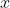miles, he increases to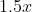miles in the second week, and decreases to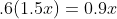for the final week -- a 10 percent decrease from his starting point.

Example Question #8 : Applying Percents To Word Problems

In a bakery, 70% of the doughnuts are glazed. If glazed doughnuts account for 21% of all pastries in the bakery, what percent of all pastries in the bakery are doughnuts?

35%

30%

28%

24%

30%

Explanation:

You can set this problem up algebraically by recognizing what you're really solving for, which is essentially a ratio of doughnuts (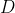) to total (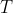). You're given that the ratio of glazed (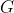) to doughnuts () is 70/100, and you're given that glazed () to total () is 21/100. Knowing that, you can set up: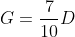and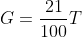And sincehas to be equal in each of those equations, you can set the equations equal: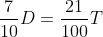From there, your goal is to solve for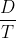, so you'll divide both sides byto get: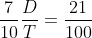And then multiply both sides by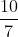to cancel the fraction on the left and move all the numbers to the right. Now you have: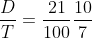Now you should see how cleanly the terms on the right factor, leaving you with: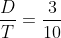. Ifout ofis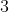out of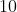, that means that the answer is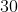.

Example Question #9 : Applying Percents To Word Problems

During a clearance sale, a retailer discounted the original price of its TVs by 25% for the first two weeks of the month, then for the remainder of the month further reduced the price by taking 20% off the sale price. For those who purchased TVs during the last week of the month, what percent of the original price did they have to pay?

60%

40%

55%

45%

60%

Explanation:

With percent problems, the key is often to make sure that you take the percent of the correct value. In this case, the initial 25% off means that customers will pay 75% of the original price. Then for the second discount, keep in mind that the discount is taken off of the sale price, not of the original price. So that's 20% off of the 75% that they did pay, which can be made easier by looking at what the customer does pay: 80% of the 75% sale price. Using fractions, that means they pay: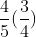of the original price, which nets to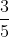of the original price, or 60%.

Example Question #10 : Applying Percents To Word Problems

A part-time employee whose hourly wage was decreased by 20 percent decided to increase the number of hours worked per week so that the employee's total income did not change. By what percent should the number of hours worked be increased?

20%

50%

25%

12.5%

We can set up equations for income before and after the wage reduction. Initially, the employee earns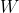wage and works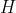hours per week. After the reduction, the employee earns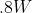wage and works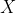hours. By setting these equations equal to each other, we can determine the increase in hours worked: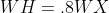(divide both sides by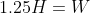We know that the new number of hours worked will be 25% greater than the original number.